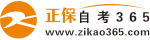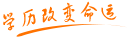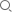#24小时客服：4008135555/010-82335555# 自考“C语言程序设计”模拟试题五答案

2007年05月28日    来源：   字体：   打印

一、填空

1.float  double

2. 0

3.ch！=‘\0’（ch>=‘a’ && ch<=‘z’）||（ch>=‘A’ && ch<=‘Z’）

4.将字符c所包含的值输出到标准的输出设备上。

5.定义一个整型的指针变量p

6.0

7.FILE  *fp

8.12

二、单项选择题

1、A     2、C     3、B     4、B     5、D     6、CD    7、D

8、B     9、D     10、C    11、A.   13、D    14、B    22、A

15、A.   16、A    17、A    18、B    19、A    20、C    21、C

三、读出下列程序，写出程序的运行结果

1.a=9，b=9

2. 28

3. f=68

4. 95

5. 8，11

四、将下列程序中不正确的语句改正

1.long   int k ；   printf （ “%ld ”， k） ；  }

2.scanf （ “%d，%d”，a ， b ）；

3.while （k < =100 ）

{sum = sum+k ；

k + + ； }

4.int  k，a ；

scanf（“%d”， &a[k]）；

5.if （a = = 1）

五、编程题

1.main（）

{ long int  a，b，sum=0，p；

for（a=1；a<=4；a++）

{ p=1；

for（b=1；b<=a；b++）

p*=b；

sum+=p；  }

printf（“%ld”，sum）；}

2、main（）

{int  a，b，c，max；

scanf（“%d，%d，%d”，&a，&b，&c）；

if （a>b）

max=a；

else

max=b；

if （max<c）

max=c；

printf（“max=%d”，max）；   }

3. int  stu（int a，int b）

{ int c；

c=a*a+b*b；

return c；     }

main（）

{   int  x，y，z；

scanf（“%d，%d”，&x，&y）；

z=stu（x，y）；

printf（“%d”，z）；    }

4.main（）

{  float  a，b，max，min，sum=0，aver；

for（b=0；b<10；b++）

scanf（“%f”，&a[b]）；

max=min=a；

for（b=1；b<10；b++）

{ if（a[b]>max）

max=a[b]；

if（a[b]<min）

min=a[b]；

sum+=a[b]；           }

sum=sum-max-min；

aver=sum/8；

printf（“%f，%f，%f”，aver，max，min）；    }

5. main（）

{   FILE  *fp；

Char ch；

fp=fopen（“write.txt”，“w”）；

ch=getchar（）；

while（ch！= ‘\n’）

{   fputc（ch，fp）；

ch=getchar（）；   }

fclose（fp）；

}

• 热门课程
• 报名咨询

• 相关问答
• 热门问答

• 购物车
• APP下载扫一扫，立即下载

安卓版本：

苹果版本：

开发者：北京正保自考教育科技有限公司

应用涉及权限：查看权限>>

APP隐私策略：查看政策>>

• 公众号扫一扫，立即关注

• 微信群进群领试题/资料